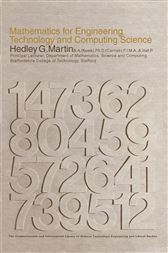Mathematics for Engineering, Technology and Computing Science

The Commonwealth and International Library: Electrical Engineering DivisionCA\$81.00
Mathematics for Engineering, Technology and Computing Science is a text on mathematics for courses in engineering, technology, and computing science. It covers linear algebra, ordinary differential equations, and vector analysis, together with line and multiple integrals.
This book consists of eight chapters and begins with a discussion on determinants and linear equations, with emphasis on how the value of a determinant is defined and how it may be obtained. Solution of linear equations and the dependence between linear equations are also considered. The next chapter introduces the reader to matrix algebra and linear equations; ordinary differential equations; ordinary linear differential equations of the second order; and solution in power series of differential equations. The Laplace transformation is also examined, along with line and multiple integrals. The last chapter is devoted to vector analysis and includes the basic ideas needed for an algebra of vectors as well as examples and problems of several applications.
This monograph will be of interest to students of mathematics, computer science, and engineering courses.

• Elsevier Science; April 2016
• ISBN: 9781483160375